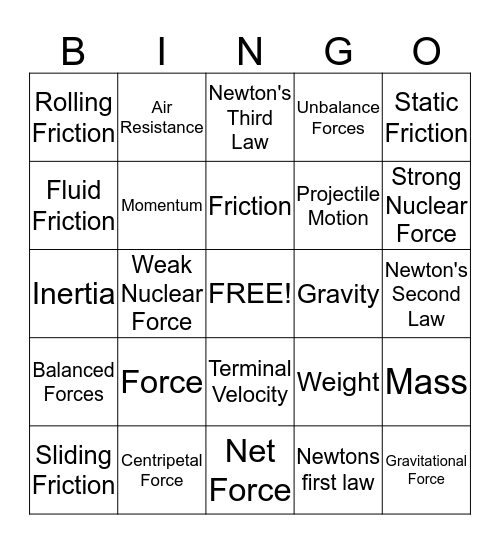# Ch.12 ForcesThis bingo card has a free space and 25 words: Force, Net Force, Friction, Sliding Friction, Static Friction, Rolling Friction, Fluid Friction, Air Resistance, Gravity, Terminal Velocity, Projectile Motion, Inertia, Weight, Mass, Momentum, Newton's 1st Law, Newton's Second Law, Newton's Third Law, Strong Nuclear Force, Weak Nuclear Force, Gravitational Force, Centripetal Force, Balanced Forces, Unbalance Forces and Newtons first law.

## Play Online